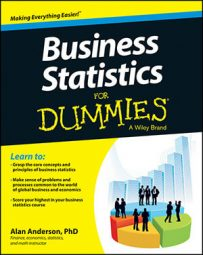Statistics make it possible to analyze real-world business problems with actual data so that you can determine if a marketing strategy is really working, how much a company should charge for its products, or any of a million other practical questions. The science of statistics uses regression analysis, hypothesis testing, sampling distributions, and more to ensure accurate data analysis.

## 3 ways to describe populations and samples

When you’re working with populations and samples (a subset of a population) in business statistics, you can use three common types of measures to describe the data set: central tendency, dispersion, and association.

By convention, the statistical formulas used to describe population measures contain Greek letters, while the formulas used to describe sample measures contain Latin letters.

### Measures of central tendency

In statistics, the mean, median, and mode are known as measures of central tendency; they are used to identify the center of a data set:

• Mean: The value between the largest and smallest values of a data set, obtained by a prescribed method.

• Median: The value which divides a data set into two equal halves

• Mode: The most commonly observed value in a data set

Samples are randomly chosen from populations. If this process is carried out correctly, each sample should accurately reflect the characteristics of the population. So, a sample measure, such as the mean, should be a good estimate of the corresponding population measure. Consider the following examples of mean:

Population mean:

This formula simply tells you to add up all the elements in the population and divide by the size of the population.

Sample mean: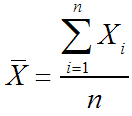The process for computing this is exactly the same; you add up all the elements in the sample and divide by the size of the sample.

In addition to measures of central tendency, two other key types of measures are measures of dispersion (spread) and measures of association.

### Measures of dispersion

Measures of dispersion include variance/standard deviation and percentiles/quartiles/interquartile range. The variance and standard deviation are closely related to each other; the standard deviation always equals the square root of the variance.

The formulas for the population and sample variance are:

Population variance: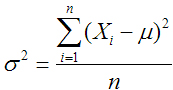Sample variance: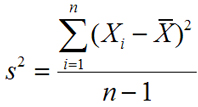Percentiles split up a data set into 100 equal parts each consisting of 1 percent of the values in the data set. Quartiles are a special type of percentiles; they split up the data into four equal parts. The interquartile range represents the middle 50 percent of the data; it’s calculated as the third quartile minus the first quartile.

### Measures of association

Another type of measure, known as a measure of association, refers to the relationship between two samples or two populations. Two examples of this are the covariance and the correlation:

Population covariance: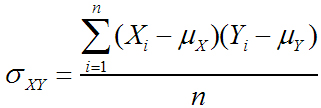Sample covariance: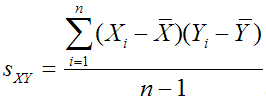Population correlation: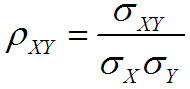Sample correlation: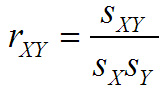The correlation is closely related to the covariance; it’s defined to ensure that its value is always between negative one and positive one.

## Random variables and probability distributions

Random variables and probability distributions are two of the most important concepts in statistics. A random variable assigns unique numerical values to the outcomes of a random experiment; this is a process that generates uncertain outcomes. A probability distribution assigns probabilities to each possible value of a random variable.

The two basic types of probability distributions are discrete and continuous. A discrete probability distribution can only assume a finite number of different values.

Examples of discrete distributions include:

• Binomial

• Geometric

• Poisson

A continuous probability distribution can assume an infinite number of different values. Examples of continuous distributions include:

• Uniform

• Normal

• Student’s t

• Chi-square

• F

## Understand sampling distributions

In statistics, sampling distributions are the probability distributions of any given statistic based on a random sample, and are important because they provide a major simplification on the route to statistical inference. More specifically, they allow analytical considerations to be based on the sampling distribution of a statistic, rather than on the joint probability distribution of all the individual sample values.

The value of a sample statistic such as the sample mean (X) is likely to be different for each sample that is drawn from a population. It can, therefore, be thought of as a random variable, whose properties can be described with a probability distribution. The probability distribution of a sample statistic is known as a sampling distribution.

According to a key result in statistics known as the Central Limit Theorem, the sampling distribution of the sample mean is normal if one of two things is true:

• The underlying population is normal

• The sample size is at least 30

Two moments are needed to compute probabilities for the sample mean; the mean of the sampling distribution equals: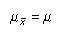The standard deviation of the sampling distribution (also known as the standard error) can take on one of two possible values: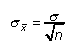This is the appropriate choice for a “small” sample; for example, the sample size is less than or equal to 5 percent of the population size.

If the sample is “large,” the standard error becomes: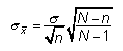Probabilities may be computed for the sample mean directly from the standard normal table by applying the following formula: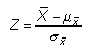## Explore hypothesis testing in business statistics

In statistics, hypothesis testing refers to the process of choosing between competing hypotheses about a probability distribution, based on observed data from the distribution. It’s a core topic and a fundamental part of the language of statistics.

Hypothesis testing is a six-step procedure:

1.    Null hypothesis

2.    Alternative hypothesis

3.    Level of significance

4.    Test statistic

5.    Critical value(s)

6.    Decision rule

The null hypothesis is a statement that’s assumed to be true unless there’s strong contradictory evidence. The alternative hypothesis is a statement that will be accepted in place of the null hypothesis if it is rejected.

The level of significance is chosen to control the probability of a “Type I” error; this is the error that results when the null hypothesis is erroneously rejected.

The test statistic and critical values are used to determine if the null hypothesis should be rejected. The decision rule that is followed is that an “extreme” test statistic results in rejection of the null hypothesis. Here, an extreme test statistic is one that lies outside the bounds of the critical value or values.

Hypotheses are often tested about the values of population measures such as the mean and the variance. They are also used to determine if a population follows a specified probability distribution. They also form a major part of regression analysis, where hypotheses are used to validate the results of an estimated regression equation.

## How businesses use regression analysis statistics

Regression analysis is a statistical tool used for the investigation of relationships between variables. Usually, the investigator seeks to ascertain the causal effect of one variable upon another — the effect of a price increase upon demand, for example, or the effect of changes in the money supply upon the inflation rate.

Regression analysis is used to estimate the strength and the direction of the relationship between two linearly related variables: X and Y. X is the “independent” variable and Y is the “dependent” variable.

The two basic types of regression analysis are:

• Simple regression analysis: Used to estimate the relationship between a dependent variable and a single independent variable; for example, the relationship between crop yields and rainfall.

• Multiple regression analysis: Used to estimate the relationship between a dependent variable and two or more independent variables; for example, the relationship between the salaries of employees and their experience and education.

Multiple regression analysis introduces several additional complexities but may produce more realistic results than simple regression analysis.

Regression analysis is based on several strong assumptions about the variables that are being estimated. Several key tests are used to ensure that the results are valid, including hypothesis tests. These tests are used to ensure that the regression results are not simply due to random chance but indicate an actual relationship between two or more variables.

An estimated regression equation may be used for a wide variety of business applications, such as:

• Measuring the impact on a corporation’s profits of an increase in profits

• Understanding how sensitive a corporation’s sales are to changes in advertising expenditures

• Seeing how a stock price is affected by changes in interest rates

Regression analysis may also be used for forecasting purposes; for example, a regression equation may be used to forecast the future demand for a company’s products.

Due to the extreme complexity of regression analysis, it is often implemented through the use of specialized calculators or spreadsheet programs.